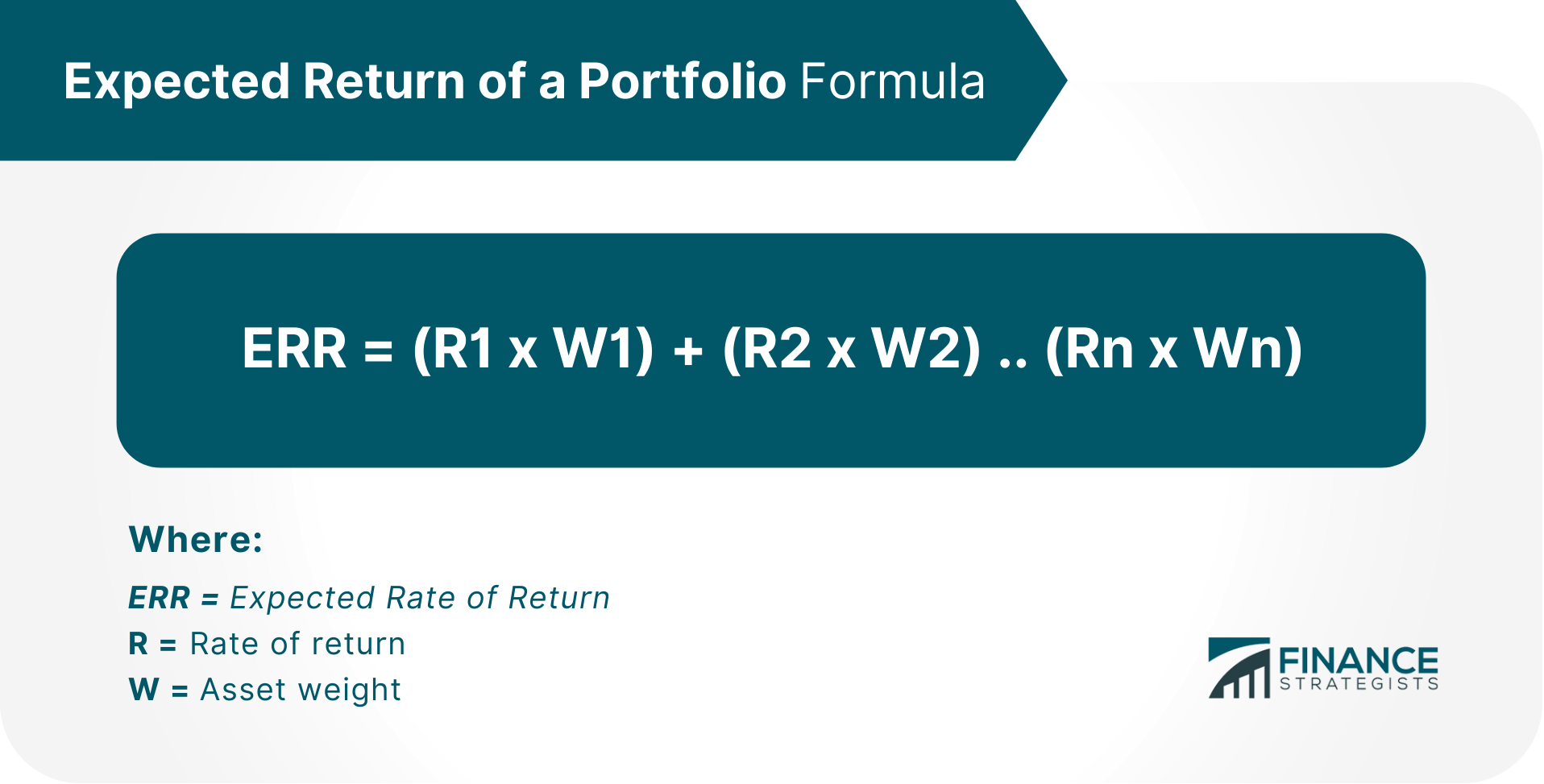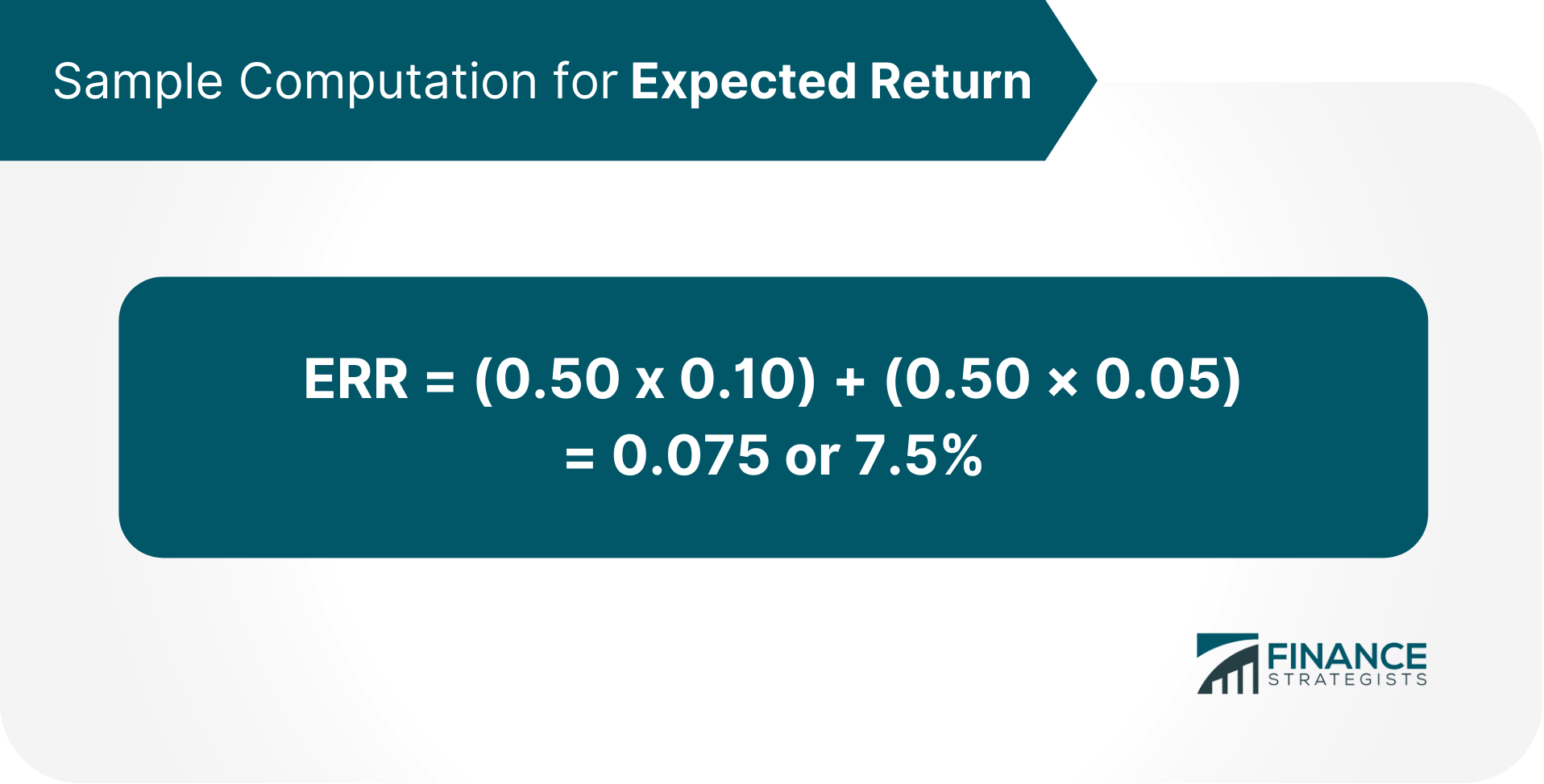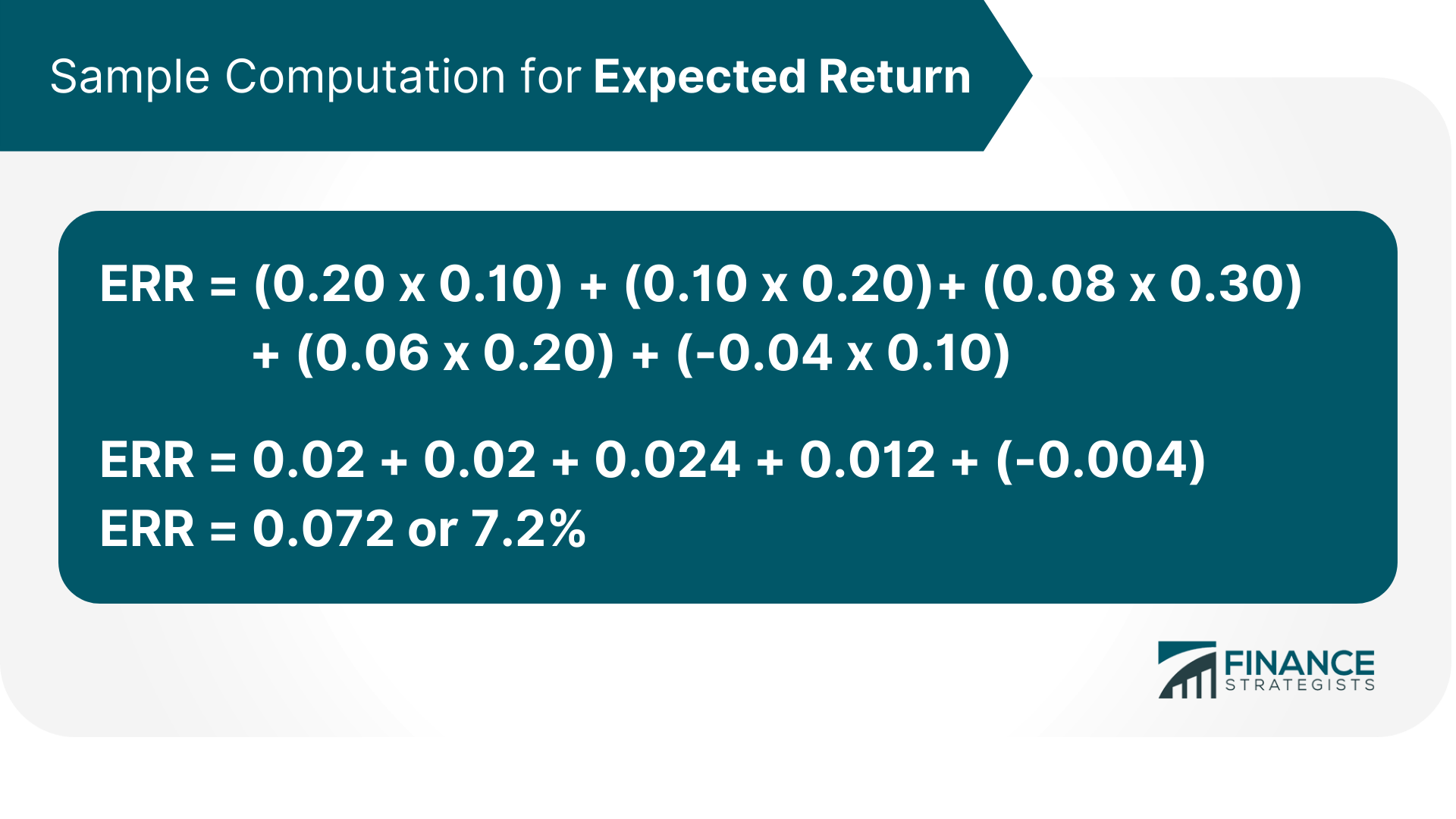# Expected Return (ER) of a Portfolio### Written byTrue Tamplin, BSc, CEPF® | Reviewed by Editorial Team

Updated on January 05, 2023

An expected return, or ER, is the return that is expected on an investment.

It is the total amount of money you can expect to gain or lose on an investment with a predictable rate of return. An expected return is often expressed in percentages, with positive returns representing profits and negative returns representing losses.

Expected returns are used in capital budgeting to compare the profitability of investments with different cash flows over time.

They are used by investors seeking to determine whether an investment is worthwhile based on their required rate of return. It allows people who are diversifying their investment portfolios to choose investments that will work best for them.

## The Formula of Expected Return of a PortfolioWhere: R = Rate of return The rate of return is a calculation that estimates the annual return on an investment over a given period. Essentially, the calculations estimate how much you would have earned or lost had you invested in some asset.

The rate of return can be either positive or negative.

W = Asset weight Asset weight is the percentage of an investment's value that is represented by a particular asset.

It is used in calculations of expected return to determine the weighted average rate of return.

## Sample Computation for Expected Return

To find the ER for a portfolio, sum up each position's ER and then weight it by the position's percentage of the portfolio.

For example, if you have a portfolio that is made up of 50% stocks and 50% bonds, and the expected return on stocks is 10%, and the expected return on bonds is 5%, then your expected return would be calculated as:The same calculation is done if you have 3 or more assets in the portfolio. Let us take another example of an investment with 5 stocks in the portfolio. The rate of return and weight for each stock are as follows: Stock A

• Rate of return = 20%
• Weight =10%

Stock B

• Rate of return = 10%
• Weight = 20%

Stock C

• Rate of return = 8%
• Weight = 30%

Stock D

• Rate of return = 6%
• Weight = 20%

Stock E

• Rate of return = -4%
• Weight = 10%

The rate of return for the entire portfolio would be calculated as follows:## Limitations of Expected Return

An expected return is not a guarantee; it is an estimate of what you can expect to earn on an investment.

Actual rates of return may be different than estimated, and you may lose money on an investment. It is also important to note that expected returns are based on historical data, and past performance is not always indicative of future results.

The estimated return for investment may change over time as the market conditions change.

In addition, because expected returns are calculated using weighted averages, they can be impacted by the inclusion or exclusion of a particular asset.

For example, if an investment has a high rate of return but is only a small part of the portfolio, its impact on the overall expected return will be limited. Therefore, it is important to use caution when interpreting and using expected returns as an indicator of investment performance.

While they provide a useful tool for comparison, they should not be the only factor considered when making investment decisions.

## The Importance of Diversification in Your Portfolio

Diversifying your investment portfolio is one of the most important things you can do to protect your investments.

When you spread your money out among different types of investments, you reduce your risk of losing money if anyone's investment performs poorly.

Diversification also helps to ensure that you will not lose all your money if a single investment goes bankrupt. Investing in a portfolio of different types of assets is one way to achieve diversification. However, it is important to remember that not all asset categories are created equal.

For example, investing in stocks and bonds is considered more conservative than investing in technology stocks.  And while real estate may be a safe investment, it is not nearly as profitable as investing in stocks and bonds.

Therefore, it is important to perform your due diligence and learn about an investment before investing in it.

This way, you will feel more confident when making investment decisions and better identify potential risks and rewards.

## Final Thoughts

An expected return is a key tool in helping you make wise investment choices.

As long as you understand the limitations of an expected return, it can be a helpful way to compare investments and see which ones are likely to provide the biggest returns over time. However, because diversification helps reduce risk and prevent losses that one investment might cause, it is important to remember that it should be one of the key factors you consider when making investment decisions.

And finally, always remember to do your own research before investing in any asset, as past performance is not always indicative of future results.

## Expected Return (ER) of a Portfolio FAQs

### What is the expected return of a portfolio?

An expected return of a portfolio is the weighted average rate of return for all the assets in the portfolio. The weights represent the proportion invested in each asset in the entire investment portfolio and can be found by simply multiplying each asset's rate of return with its corresponding percentage.

### How to calculate the expected return of a portfolio?

The expected return of a portfolio is the sum of all the assets' expected returns, weighted by their corresponding proportion.

### Formula of Expected Return of a Portfolio:

Expected Rate of Return (ERR) = (R1 x W1) + (R2 x W2) .. (Rn x Wn) Where R is the rate of return and W is the asset weight.

### What asset classes should I include in my portfolio for optimal diversification?

This is a difficult question to answer as it depends on numerous factors, such as an investor's age, risk tolerance, and investment goals. Generally speaking, it is recommended that an investor have a diverse portfolio that includes stocks, bonds, real estate, and cash equivalents.

### Why is diversification important?

Diversification is important because it helps to reduce an investor's risk by spreading their money out among different types of investments. When you invest in a portfolio of different types of assets, you are less likely to lose money if anyone's investment performs poorly.